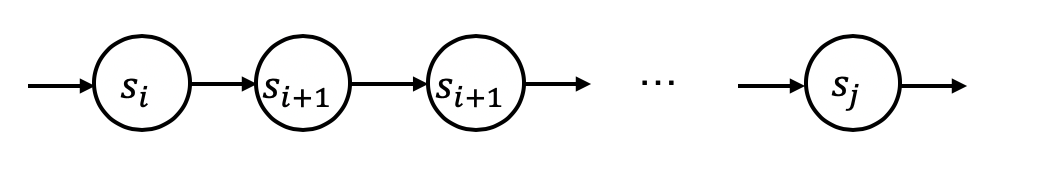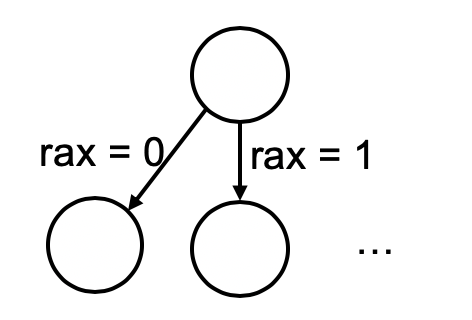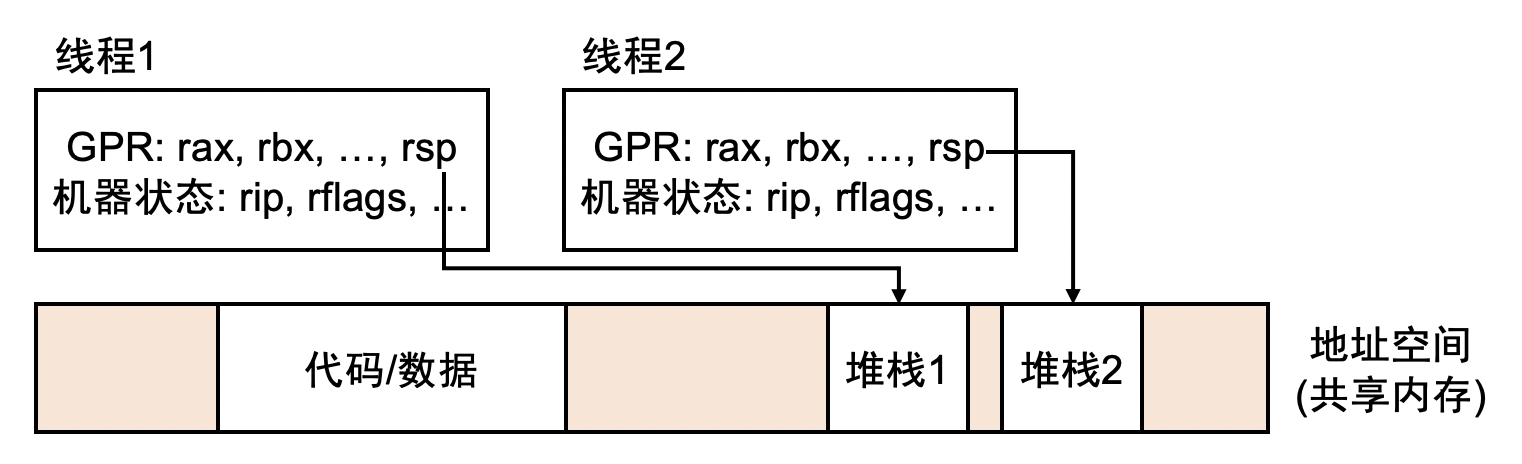# 共享内存多线程

## 1. 状态机

“状态机” 是对物理世界运行的抽象数学模型。熟悉图论的同学，不妨把状态机看成是有向图 $G(V,E)$，其中：

• 图的每个节点 $s \in V$ 代表一个 “状态”，不同的节点通常代表不同的状态，例如下图是一个 (可怜的、没有娱乐的) 人状态的抽象——他要么在工作，要么在家，要么在床上：• 图中的边 $(s_1, s_2) \in E$ 代表状态的转换，有时也写作 $s_1 \to s_2$。当状态机系统的外部发生变化时，状态机的状态随之会发生改变，例如当前状态是 “在家”，那么既有可能进入 “床上” 的状态睡觉，也有可能乘地铁上班，进入 “工作” 的状态。

• 图中的边有时有标签 (label)，表示系统外界发生的具体变化。

1. 状态机是物理世界的抽象，几乎任何物理对象都或多或少可以写成状态机。一旦状态机建立，我们就得到了一个图——于是我们可以用图论的算法去研究状态机的性质。例如，当我们写出一个并发程序 (并发算法、并发协议) 的状态机后，就能用图论的语言定义并发程序算法的 “正确性”，并在 $|E|$ 的多项式时间内完成对算法的证明。
2. 计算机系统的本质天然是数字逻辑电路实现的状态机，因此状态机模型也可以帮助我们理解计算机系统的方方面面。状态机的视角帮助我们理解处理器设计、程序调试、自动测试等 non-trivial 的计算机系统解决方案。

## 2. 程序的状态机模型

• CPU 根据当前的 PC 指针 (例如 x86-64 的 rip 寄存器)，在内存中取出一条指令；
• 对指令进行译码、执行，指令将按照手册上约定的行为，修改 CPU 的寄存器或内存。

(gdb) info registers   // 查看寄存器
rax            0x55555555463a   93824992233018
rbx            0x0  0
...
rip            0x55555555463e   0x55555555463e <main+4>
eflags         0x246    [ PF ZF IF ]
...
(gdb) x/16i 0x55555555463e   // 查看内存
=> 0x55555555463e <main+4> :  lea    0x9f(%rip),%rdi        # 0x5555555546e4
0x555555554645 <main+11>:  callq  0x555555554510 <puts@plt>
0x55555555464a <main+16>:  mov    $0x0,%eax 0x55555555464f <main+21>: pop %rbp 0x555555554650 <main+22>: retq ...  如果使用单步执行一条指令，我们将会看到状态的变化，而且状态的变化符合是 rip 寄存器位置指令的行为。因此，我们不妨把所有的寄存器/内存的数值作为程序的状态，程序在两个时刻寄存器/内存数值只要有任何不同，就认为是两个不同的状态。 程序每执行一条指令，状态就会发生变化 (指令执行的结果)。我们用$(M,R)$来表示程序的状态，其中$M$是程序所有内存的数值；$R$是程序所有寄存器的数值。例如，假设$M[R[\textrm{rip}]]$的字节序列是 48 c7 c0 01 00 00 00，即 movl$1, %rax。那么，执行这条指令后程序的状态是 $(M',R')$，则有：

$R'[\textrm{rip}] = R[\textrm{rip}] + 7$ (下一条指令位于 7 个字节之后), $R'[\textrm{rax}] = 1$ (rax 寄存器被设置为 1)

### 2.1. 确定 (deterministic) 和不确定 (non-deterministic) 的指令#include <stdio.h>
#include <stdint.h>

int main() {
uint64_t val;
asm volatile ("rdrand %0": "=r"(val));
printf("rdrand returns %016lx\n", val);
}1. 根据读入流的情况，有可能返回 -1 (失败)、0 (end of file)、某个正数代表读取的字节数；
2. read 传入的 buf 可能会被操作系统修改为读取到的数据。

## 2.2. 并发程序的状态机模型

$(M, R_1, R_2, \ldots, R_n)$• 选择线程 $1$ 执行：$(M, R_1) \to (M_1', R_1')$，得到状态 $(M_1', R_1', R_2, \ldots, R_n)$；
• 选择线程 $2$ 执行：$(M, R_2) \to (M_2', R_2')$，得到状态 $(M_2', R_1, R_2', \ldots, R_n)$；
• $\ldots$
• 选择线程 $n$ 执行：$(M, R_n) \to (M_n', R_n')$，得到状态 $(M_n', R_1, R_2, \ldots, R_n')$；$./hello-mt.out &  18401$ pmap $! #$! 是上一个后台进程的 pid
18401:   ./hello-mt.out
00005629379d2000      4K r-x-- hello-mt.out
0000562937bd2000      4K r---- hello-mt.out
0000562937bd3000      4K rw--- hello-mt.out
00005629387e7000    132K rw---   [ anon ]
00007fde98000000    132K rw---   [ anon ]
00007fde98021000  65404K -----   [ anon ]
00007fde9dd7c000      4K -----   [ anon ]
00007fde9dd7d000   8192K rw---   [ anon ]
00007fde9e57d000      4K -----   [ anon ]
00007fde9e57e000   8192K rw---   [ anon ]


#### 动手实践

int *shared;

int x;
...
shared = &x; // 局部变量的地址
...
}
...
*shared = 1; // 向另一个线程的堆栈中写入输入；
// 只要 thread1() 不返回，这个写入就是合法的
...
}


$n$ 个线程的并发程序，若每个线程执行 $m$ 条指令，就算所有指令都是确定的，不同的执行顺序也多达 $n^{O(mn)}$，这根本就是个天文数字，也难怪并发程序的执行难理解了——冷不丁的犄角旮旯里就藏着并发的 bug。

### 2.3. 理解并发程序的执行

int turn, x = 0, y = 0;

 x = 1;
 turn = T2;
 while (y && turn == T2) ;
 // critical section
 x = 0;
}

 y = 1;
 turn = T1;
 while (x && turn == T1) ;
 // critical section
 y = 0;
}


#### 思考题：为什么多此一举？

Peterson 算法中有三个变量，对应了现实中 $T_1$ 的旗子、$T_2$ 的旗子和一块牌子。这似乎是必不可少的——你可以试试如果只用旗子的互斥，例如：

void thread1() {
x = 1;
while (y);
}
y = 1;
while (x);
}


void thread1() {
retry:
x = 1;
if (y) x = 0;
if (!x) goto retry;
}
retry:
y = 1;
if (x) y = 0;
if (!y) goto retry;
}


Peterson 算法 (以及其他并发算法) 的正确性分为两个部分：

### 2.3.2: 证明好事永远会发生 (Liveness)

“好事总会发生” 的定义稍稍困难一些。我们将它表达为，“如果给两个线程足够的时间，一定至少有一个能进入临界区”，即到达 $\textrm{PC}_1 = 4 \lor \textrm{PC}_2 = 4$。

Liveness 定义的困难之处在于，我们要给 “两个线程足够的时间”。只给一个线程足够的时间是不够的——它自己可能无限循环，但只要让它和另一个线程交替执行几步，就可以进入临界区。

#### 图论的语言和算法

“不存在” 性的证明通常求解它的反面：即我们只要求出一条不满足 liveness 的无穷路径，就能证明 liveness 被违反了。无穷路径怎么求呢？这里用一点算法上的小 trick：

• 一条无穷长的路径、$T_1$, $T_2$ 都出现了无穷多次，当且仅当 $T_1$, $T_2$ 交替出现无穷多次。

“程序就是状态机” 是一个非常强大的工具，能够帮助我们理解程序的执行，这也是操作系统课程上所用为数不多的理论工具之一。

## 3. 实现一个 Model Checker

### 3.1. 设计 Model Checker

_init:
x: 0
y: 0
turn: X


T1: |
x = 1
turn = 'T2'
if y != 1: GOTO(4)
if turn == 'T2': GOTO(2)
x = 0
GOTO(0)
T2: |
y = 1
turn = 'T1'
if x != 1: GOTO(4)
if turn == 'T1': GOTO(2)
y = 0
GOTO(0)


_bug_on: |
PC['T1'] == 4 and PC['T2'] == 4 # 在两个线程同时进入临界区时标记错误
_mark_on: |
PC['T1'] == 4 or PC['T2'] == 4  # 在至少有一个线程进入临界区时标记提醒


### 3.2. 实现 Model Checker

queue, explored, nodes, edges = [ s0 ], { H(s0) }, [ s0 ], []
while queue:
s, queue = queue, queue[1:]
s1 = expand(s, t)
if H(s1) not in explored:
nodes.append(s1)
queue.append(s1)
edges.append((H(s), H(s1), t))


Model checker 实现中第一个有趣的 hack 是我们对代码的预处理。Python 中并没有 GOTO——我们是如何表达线程状态 (PC) 的变化的呢？短短几行的程序：

def parse(code):
for line in code.strip().splitlines():
yield re.sub(r'GOTO$$(.*?)$$', r'pc = \1 - 1', line.strip() + '\npc += 1')


turn = 'T2'
pc += 1


if y != 1: GOTO(4) 则会被替换为

if y != 1: pc = 4 - 1
pc += 1 # 因为 pc += 1 是每次都添加的，所以 GOTO 的目标减去了 1


def expand(s, t):
pc   = s['PC'][t]
stmt = P[t][pc]
s1   = { **s, 'PC': s['PC'].copy(), 'pc': pc }
exec(stmt, {}, s1) # hah! we're cheating!
s1['PC'][t] = s1['pc']
return { k: s1[k] for k in variables }


Peterson 算法的状态树，只显示到达每个状态的最短路径树。

## 4. 延伸阅读

• 给一次程序的执行 ($s_0 \to s_1 \to\ldots$)，如果记录下所有的状态 $s_i$，我们自然可以随时查看 $s_i$ 的状态，从而实现 “时间旅行”。但这样记录状态的代价太大了——当然，我们知道通常 $s_0$ 的状态是容易记录的；由于每条指令的执行通常只影响数量很少的寄存器和内存，我们可以记录 $s_i$ 和 $s_{i+1}$ 之间 “不同” 的部分，就像 diff，我们就可以像给程序 “打补丁” 一样自由地向前或向后执行。
• 如果我们对 “时间穿梭” 没有兴趣，只是想重现一次程序执行的结果。假设所有指令都是确定的，那么只要记录下 $s_0$，无论执行多少次，都必然得到完全相同的执行结果。对于应用程序来说，产生不确定性的指令数量是很少的 (rdrand, syscall 等)。因此，只需要在这些指令之后记录下指令带来的不确定后果，例如：

• 对于 rdrand，记录下得到的随机数值；
• 对于 syscall，记录下系统调用返回的结果，例如 read 需要记录它的返回值和读到的数据。

然后，我们只要在重放 (replay) 时，遇到这些不确定的指令时不实际执行，而是用记录下的结果替代，就能实现程序行为的精准重放。同理，也可以实现整虚拟机完整执行的记录和重放。事实上，很多游戏模拟器就内置了这样的记录功能。有兴趣的同学可以阅读推荐的论文：